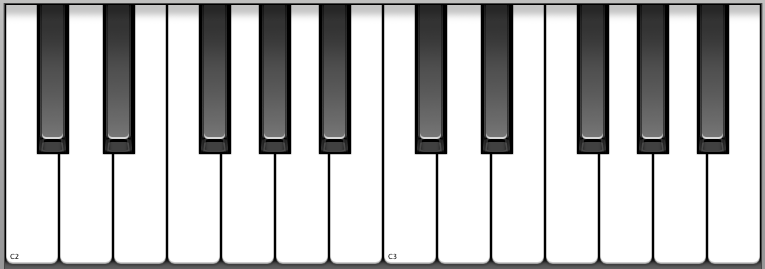# Hamden High School Department of Fine Arts Mr. Hicks Major and Minor Triads I intervals

 Hamden High School Department of Fine Arts Mr. Hicks Major and Minor Triads I INTERVALS Musicians use the term interval to show the distance between two pitches. A half step is the interval minor second (written m2) and a whole step is an interval of a major second (written M2). All seconds use notes that are next to each other in the alphabet (such as AB, BC. CD, etc.). The distance from A to C is a third, because C is the third letter counting up from A.Look at the keyboard and count the half steps from A to C. You will find that the distance from A to C is three half steps, and so it is known as a minor third (m3). Now count the half steps from C to E. You will find that this interval is four half steps, so it is known as a major third (M3). See the following list of thirds and notice that some are major and some are minor, depending on whether the distance between the pitches is three or four half steps. AC = m3 BD = m3 CE = M3 DF = m3 EG = m3 FA = M3 GB = M3 The other interval that you need to know for triads is the fifth. Fifths come in three sizes: diminished (small), perfect (medium), and augmented (large). Most triads use the interval of a perfect fifth (P5) from the root to the fifth of the triad. A perfect fifth is seven half steps, a diminished fifth (d5) is six half steps, and an augmented fifth (A5) is eight half steps. See the following list of fifths. AE = P5 BF = d5 CG = P5 DA = P5 EB = P5 FC = P5 GD = P5 TRIAD THEORY Root position triads are chords consisting of three pitches that use alternate letters of the music alphabet. The basic triads of the musical alphabet are ACE, BDF, CEG, DFA, EGB, FAC, GBD. The first letter of a triad is known as the root, the middle letter is the third, and the last letter is the fifth. There are four possible combinations of intervals within triads. When the interval from the root to the third is a major third (M3) and the interval from the root to the fifth is a perfect fifth (P5) the triad is major (such as CEG, FAC, and GBD). When the interval from the root to the third is a minor third (m3) and the interval from the root to the fifth is a perfect fifth (P5) the triad is minor (such as ACE, DFA, and EGB). When the interval from the root to the third is a minor third (m3) and the interval from the root to the fifth is a diminished fifth (d5) the triad is diminished (such as BDF). When the interval from the root to the third is a major third (M3) and the interval from the root to the fifth is an augmented fifth (A5) the triad is augmented. No accidentals occur in the natural musical alphabet (sharp, flat, or natural signs are needed). BASIC TRIADS: GBD FAC EGB DFA CEG BDF ACE All triads are based on the seven triads listed above. Memorize them! The following symbols are used for the four qualities of triads: C = C major triad Cm = C minor triad (also C-) Cº = C diminished triad (also seen as Cdim) C+ = C augmented (also seen as Caug) TRIADS ON THE KEYBOARD Commonly Used Major Triads CEDDownload 390.35 Kb.Share with your friends: